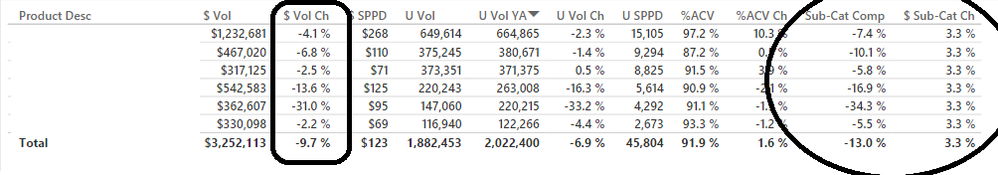cancel
Showing results for
Did you mean:

Fabric is Generally Available. Browse Fabric Presentations. Work towards your Fabric certification with the Cloud Skills Challenge.Resolver I

## ALLEXCEPT only working on visible row context

Hello,

I have a quick question. I am trying to calculate the \$ Change (YOY) for a sub category, and then trying to compare that overall sub category change to specific products. Basically, we want to find products that are underperforming a sub category, but by using dynamic comparison. Here is the measure I am working on:

`CALCULATE( [\$ Vol Ch], ALLEXCEPT ( Data, Data[Date], Data[Market], Data[Sub-Cat] ) )`

This works in a table or matrix if the "Sub-Cat" is being filtered or visible in the table (providing row context). However, I do not want to show the Sub-Cat in the table, because it can be assumed based on the product description (which is visible). So how can I change the measure to consider a 'non-visible row context'? Each product is already tied to a Sub-Category in the data (same data table, different column). Here is a screenshot of the data table with and without the sub-category visible:

Without sub category visible, numbers are incorrectWith sub category in table, numbers are correctThank you in advance and look forward to meeting everyone at the Data Insights Summit!

Bobby

1 ACCEPTED SOLUTIONResolver I

Have you ever posted a question and then answered it immediately afterwards?

Here is the solution:

`CALCULATE( [\$ Vol Ch], ALLEXCEPT ( Data, Data[Date], Data[Market], Data[Sub-Cat] ), VALUES( Data[Sub-Cat] ) )`
5 REPLIES 5Resolver I

Have you ever posted a question and then answered it immediately afterwards?

Here is the solution:

`CALCULATE( [\$ Vol Ch], ALLEXCEPT ( Data, Data[Date], Data[Market], Data[Sub-Cat] ), VALUES( Data[Sub-Cat] ) )`New Member

I found this post after a 4 hour struggle, thank you.Resident Rockstar

Note, there is no row context in a table visual or a matrix visual. Row context only exists in a physical data model table (imported or calculated), and in a small subset of functions, the most common of which are FILTER(), *X() functions, and ADDCOLUMNS().

The reason that your original construction doesn't work is that there is no filter context on [Sub-Cat] in the matrix that doesn't include this field as a label.

VALUES() is evaluated in filter context, so your VALUES( 'Data'[Sub-Cat] ) is evaluated in the filter context of a given [Product Desc]. The result of this VALUES() is combined with a logical and with your ALLEXCEPT(). You should be able to remove 'Data'[Sub-Cat] from the ALLEXCEPT() and get the same result.Resolver I

That makes a lot of sense. Thank you for your help!Resident Rockstar

A really good test to determine if you're in row context or not is to make a bare column reference - e.g. 'Table'[Field] not contained in any function. If the expression evaluates without the "Value for column cannot be determined in the current context..." error, then you're in a row context. If you do get that error on a bare column reference, then you're in a filter context.

E.g.

```// DAX
// Calculated Column
NewColumn =
'Table'[OtherColumn]
// The above works - row context means there's only one value for that
// field

// Measure
NewMeasure =
'Table'[NewColumn]
// The above will fail, because a filter context cannot syntactically
// guarantee a single value for that field. See below.

//Measure
CalculateNewMeasure =
CALCULATE(
'Table'[NewColumn]
,'Table'[PrimaryKey] = 1
)
// The above will fail, because CALCULATE() creates a filter context.
// Even though we know logically that the [PrimaryKey] field will only
// identify a single row of 'Table', this is not something that can be
// inferred solely from the syntax of the statement.```

The difference is what we, as model authors and intelligent human beings, can infer from the content of an expression, vs what the DAX interpreter can logically deduce only from the form of the expression.

A filter context can be created that we know to have a single row for a given field. Given that filter context, it is possible to alter the underlying data such that there will be multiple rows in the table that meet that filter context.

You might object and say that the following should work:

```// DAX
// Measure
MeasureWithSingleValue =
CALCULATE(
'Table'[Field]
,'Table[Field] = 1
)```

In this case, our expression to evaluate and our filter are both referencing the same field. There is still no aggregation around 'Table'[Field], and there might  be multiple rows that have [Field] = 1. Even if the field is our primary key, there is no syntactic way to derive that in DAX.

The below variation will return a value, either blank or 1, depending on the existence of a 1 in [Field]:

```// DAX
// Measure
MeasureWithSingleValue2 =
CALCULATE(
VALUES( 'Table'[Field] )
,'Table'[Field] = 1
)```

What's the difference? VALUES() returns distinct values from a field. Now, even if there are many rows with [Field] = 1, we've logically grouped those to a single value with VALUES().Announcements#### Power BI Monthly Update - November 2023

Check out the November 2023 Power BI update to learn about new features.#### Fabric Community News unified experience

Read the latest Fabric Community announcements, including updates on Power BI, Synapse, Data Factory and Data Activator.#### The largest Power BI and Fabric virtual conference

130+ sessions, 130+ speakers, Product managers, MVPs, and experts. All about Power BI and Fabric. Attend online or watch the recordings.Top Solution Authors
Top Kudoed Authors
Users online (2,041)